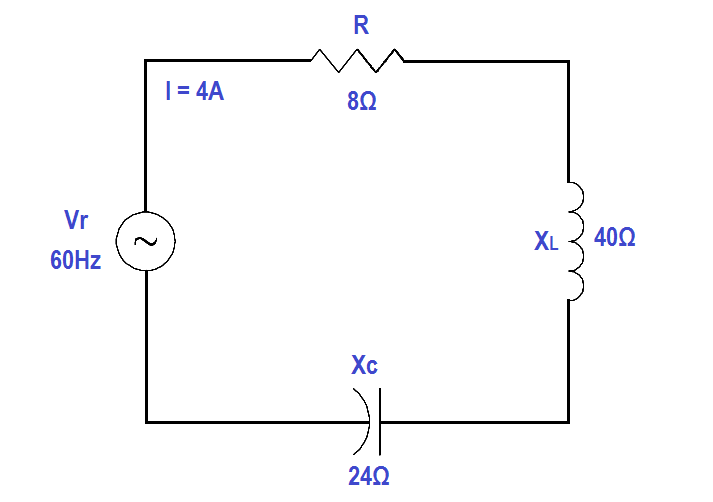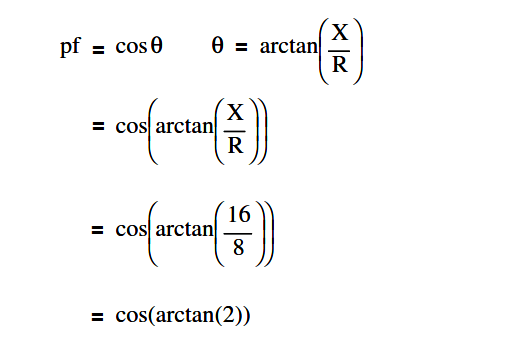# Calculate Power in Series RCL Circuit

An 8 Ω resistance, a 40 Ω XL, and a 24 Ω XC are in series with a 60 Hz source with a current flow of 4 amps, as shown in Figure.Figure : Series R-C-L Circuit

Find:

1. Z
2. Voltage, VT
3. Power Factor, pf
4. True Power, P
5. Reactive Power, Q
6. Apparent Power, S

Solution:

##### 1. Calculate Z

Z = √R2 + ( XL  –  XC )2

Z = √82 + ( 40  –  24 )2

Z = 17.9Ω

V= I Z

V= 4 x 17.9

V= 71.6 volts

##### 3. Calculate Power factor, p.f.p.f. = cos (63.4º)

p.f. = 0.45

##### 4. Calculate True Power, P

P = EI cos θ

P = (71.6)(4)(0.45)

P = 128.9 watts

##### 5. Calculate Reactive Power, Q

Q = EI sin θ

Q = (71.6)(4)(0.89)

Q = 254.9 VAR

S = EI

S = (71.6)(4)

S = 286.4 VA